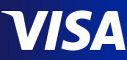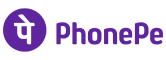New update is available. Click here to update.
Topics

# Max GCD Pair

Moderate0/80
Average time to solve is 35m+4 more companies

## Problem statement

You are given an array of positive integers. Find the GCD(Greatest Common Divisor) of a pair of elements such that it is maximum among all possible pairs. GCD(a, b) is the maximum number x such that both a and b are divisible by x.

``````For example:
If the given array is {1, 5, 2, 3, 4}, the output will be 2 (which is GCD of 2 and 4, all other pairs have a GCD of 1)
``````
Detailed explanation ( Input/output format, Notes, Images )
Constraints :
``````1 <= T <= 10
2 <= N <= 10 ^ 4
1 <= ARR[i] <= 10 ^ 5

Where 'T' is the number of test cases, 'N' is the size of the array and ARR[i] is the ith element in the array.

Time Limit: 1 sec.
``````
Sample Input 1:
``````3
5
5 2 4 3 1
5
2 4 6 5 6
10
4 2 10 8 6 4 3 2 1 7
``````
Sample Output 1:
``````2
6
4
``````
Explanation for Sample Input 1:
``````In the 1st test case, pair (2,4) has a maximum GCD value of 2, all other pairs have a GCD of 1.

In the 2nd test case, pair (6,6) has a maximum GCD value of 6, all other pairs have GCD less than 6.

In the 3rd test case, pair (4,8) has a maximum GCD value of 4, all other pairs have GCD less than 4.
``````
Sample Input 2:
``````2
4
2 4 6 8
5
1 2 3 4 5
``````
Sample Output 2:
``````4
2
``````Console